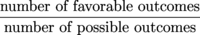Study Guide

# Probability and Statistics - In the Real World

## In the Real World

As for the statistics part, you can actually make a living out of doing statistics. In fact, there are even statistics about statisticians.

As for probability, here's a fun yet oddly practical subject, depending on what you do in your spare time. Probabilities are what determine how good your poker hand is. Of course, we know you're only playing for Werther's Originals with your grandmother and not in some seedy, underground club. Even still, knowing probabilities can help you clean out your nana's stash of sweets.

### Sample Problem

What's the probability that a five-card poker hand contains four of a kind?

The probability that the hand contains four of a kind is.

First, the numerator:

A favorable outcome is any hand that contains four of a kind. Depending on how many Werther's Originals are in the pot, it could be a very favorable outcome indeed. Don't forget...our cut is 20%.

There are 13 different kinds we could get four of (four aces, four 2s, four 3s, and so on.). Once we get four of a kind in our hand, we've already filled 4 of the 5 spaces. There are

52 – 4 = 48

cards left, and we could have any of those. That means there are

13 × 48 = 624

possible hands containing four of a kind.

Next, the denominator:

The number of possible outcomes is the total number of possible five-card poker hands. Since we don't care about the order of the cards, this is a combination question. Given 52 cards, how many ways can we choose five of them? The answer is:Now we've got the top and bottom of our fraction. The probability of a five-card poker hand containing four of a kind is,

which is a pretty tiny number. You wouldn't think so from watching poker scenes in movies, where someone seems to have four of a kind every other hand. Ah, Hollywood. Reflecting real life since 1902.

For more fun with poker, you and your nana should head over here.

• ### I Like Abstract Stuff; Why Should I Care?

Mathematicians deal with two different kinds of numbers.

Discrete numbers are numbers that have obvious gaps between them, like Letterman's teeth. For example, integers are discrete: there's a huge, obvious gap between the numbers 1 and 2, and there are no other integers between them.

Continuous numbers are numbers where no matter how close two of them are, there's another number between them. The real numbers are continuous. Even if we get two numbers as close together as 0.111 and 0.1111, we can still find a number in between them: for example, 0.11105.

Our study of probability has been dealing with discrete numbers (the number of marbles in a jar, or socks in a drawer), but mathematicians also study probabilities involving continuous numbers. If you have 0.111 socks in your drawer, it's time to go sock shopping.

If you take a data set and make histograms with thinner and thinner rectangles, you see pictures that are getting increasingly closer to something called a probability distribution, which is usually studied in calculus and beyond. Now that we've planted that irresistible little seed of anticipation in your noggin, let's wrap this puppy up.

• ### How to Solve a Math Problem

There are three steps to solving a math problem.

1. Figure out what the problem is asking.

2. Solve the problem.

### Sample Problem

Lia has 15 books but only likes two-thirds of them. If she picks two books at random to take on a plane ride, what's the probability that she will like both of the books she picks? Note to Lia: have a yard sale when you go back home.

1. Figure out what the problem is asking.

This problem is asking about "picking" or "choosing" books. Since nothing is said about order, the problem is asking about combinations rather than permutations. We're also trying to find a probability.

2. Solve the problem.

What's the probability that Lia likes both books she picks? The formula we remember for probability is.

Let's look at the number of possible outcomes first. Lia is picking two books at random. The number of ways to do this, if we don't care about order, isAn outcome is "favorable" if Lia likes both the books she picks. She likes two-thirds, or 10, of her books. If both the books she chooses come from these 10, then the outcome is favorable. The number of ways to choose her 2 books from the 10 she likes isPutting it all together, the probability Lia likes both her books is.

If she winds up with two books she doesn't like, her temperament on that plane will probably be a little...shall we say...turbulent.

Since, we can at least be sure we've found a probability. It's all about the small victories.